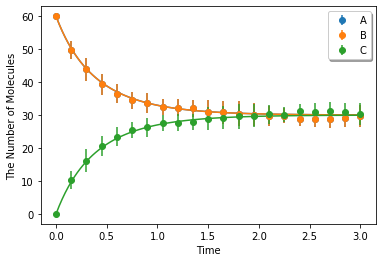# Reversible¶

This is for an integrated test of E-Cell4. Here, we test a simple reversible association/dissociation model in volume.

In :
%matplotlib inline
from ecell4.prelude import *


Parameters are given as follows. D, radius, N_A, U, and ka_factor mean a diffusion constant, a radius of molecules, an initial number of molecules of A and B, a ratio of dissociated form of A at the steady state, and a ratio between an intrinsic association rate and collision rate defined as ka andkD below, respectively. Dimensions of length and time are assumed to be micro-meter and second.

In :
D = 1
N_A = 60
U = 0.5
ka_factor = 0.1  # 0.1 is for reaction-limited

In :
N = 20  # a number of samples


Calculating optimal reaction rates. ka and kd are intrinsic, kon and koff are effective reaction rates.

In :
import numpy
kD = 4 * numpy.pi * (radius * 2) * (D * 2)
ka = kD * ka_factor
kd = ka * N_A * U * U / (1 - U)
kon = ka * kD / (ka + kD)
koff = kd * kon / ka


Start with no C molecules, and simulate 3 seconds.

In :
y0 = {'A': N_A, 'B': N_A}
duration = 3
opt_kwargs = {'legend': True}


Make a model with effective rates. This model is for macroscopic simulation algorithms.

In :
with species_attributes():

with reaction_rules():
A + B == C | (kon, koff)

m = get_model()


Save a result with ode as obs, and plot it:

In :
ret1 = run_simulation(duration, y0=y0, model=m)
ret1.plot(**opt_kwargs)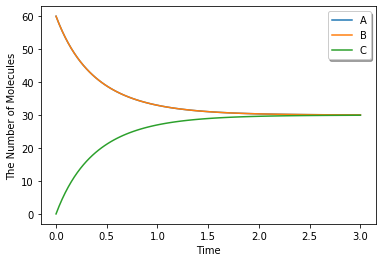Simulating with gillespie (Bars represent standard error of the mean):

In :
ret2 = ensemble_simulations(duration, ndiv=20, y0=y0, model=m, solver='gillespie', repeat=N)
ret2.plot('o', ret1, '-', **opt_kwargs)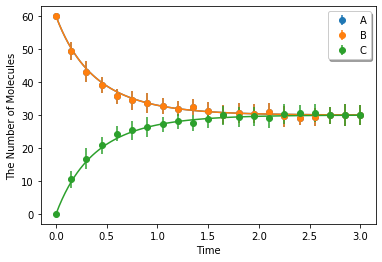Simulating with meso:

In :
ret2 = ensemble_simulations(duration, ndiv=20, y0=y0, model=m, solver=('meso', Integer3(4, 4, 4)), repeat=N)
ret2.plot('o', ret1, '-', **opt_kwargs)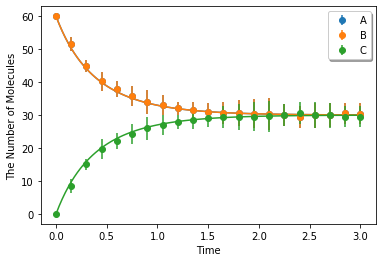Make a model with intrinsic rates. This model is for microscopic (particle) simulation algorithms.

In :
with species_attributes():

with reaction_rules():
A + B == C | (ka, kd)

m = get_model()


Simulating with spatiocyte. voxel_radius is given as radius:

In :
ret2 = ensemble_simulations(duration, ndiv=20, y0=y0, model=m, solver=('spatiocyte', radius), repeat=N)
ret2.plot('o', ret1, '-', **opt_kwargs)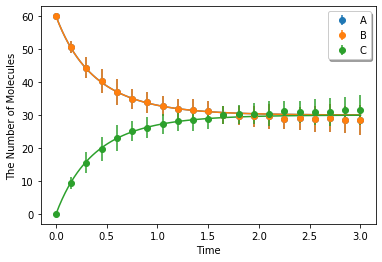Simulating with egfrd:

In :
ret2 = ensemble_simulations(duration, ndiv=20, y0=y0, model=m, solver=('egfrd', Integer3(4, 4, 4)), repeat=N)
ret2.plot('o', ret1, '-', **opt_kwargs)